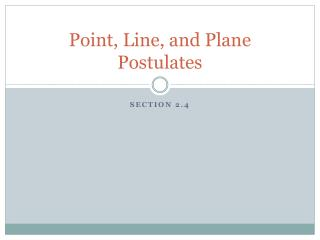DownloadDownload PresentationPoint, Line, and Plane Postulates

# Point, Line, and Plane Postulates

Télécharger la présentation## Point, Line, and Plane Postulates

- - - - - - - - - - - - - - - - - - - - - - - - - - - E N D - - - - - - - - - - - - - - - - - - - - - - - - - - -
##### Presentation Transcript

1. Point, Line, and Plane Postulates Section 2.4

2. Note-Taking Guide I suggest only writing down things in red

3. Review of Postulates from Chapter 1 • Postulate 1 = Rule Postulate • Basically you can measure length/distance with a ruler • Postulate 2 = Segment Addition Postulate • If is between and then • Postulate 3 = Protractor Postulate • Basically you can measure angles with a protractor • Postulate 4 = Angle Addition Postulate • If is in the interior of then

4. New Postulates in Section 2.4 More statements about points, lines, and planes we accept without having to prove them The reason we are learning these is to eventually use them to prove other things

5. Plane, Line, and Point Postulates Postulate 5 Through any two points there exists exactly one line

6. Plane, Line, and Point Postulates Postulate 6 A line contains at least two points

7. Plane, Line, and Point Postulates Postulate 7 If two lines intersect, then their intersection is exactly one point

8. Plane, Line, and Point Postulates Postulate 8 Through any three noncollinear points there exists exactly one plane

9. Plane, Line, and Point Postulates Postulate 9 A plane contains at least three noncollinear points

10. Plane, Line, and Point Postulates Postulate 10 If two points lie in a plane, then the line containing them lies in the plane

11. Plane, Line, and Point Postulates Postulate 11 If two planes intersect, then their intersection is a line

12. Definition Definition of Perpendicular Figures: A line is to a plane if and only if the line is to every line in the plane that it intersects Notice how line is to line and line and any other line we could draw in plane

13. Interpreting Diagrams • What stuff are we allowed to assume in this diagram? • Coplanar points • Points on drawn inlines are collinear

14. Interpreting Diagrams • What stuff are we NOT allowed to assume in this diagram? • Points without drawn in lines are collinear • Ex: G, F, E • Coplanar lines intersect • Coplanar lines do not intersect • Ex: we do not know if and intersect, but we do not know that they don’t intersect • Congruency • Perpendicular

15. Boardwork Find a marker and a spot at the board

16. True or False Two planes intersect in exactly one point. False

17. True or False A plane contains at least 3 noncollinear points. True

18. True or False Through any two points there exists exactly one line. True

19. True or False If two points are on a plane, then the line containing those points is off of the plane. False

20. True or False If two lines intersect, then their intersection is exactly two points. False

21. True or False A line is made up of exactly two points. False

22. Practice Problems • T or F: is in plane • True • State the intersection of plane and plane • T or F: and intersect • True • T or F: and intersect • False • T or F: and intersect • False (there is not enough information to assume one way or the other, so since there the potential for the statement to be false, the statement is not true 100% of the time and thus the correct answer is False)

23. Practice Problems • T or F: plane exists • True • T or F: are coplanar • False (it could potentially be false, so answer cannot be true 100% of the time, so correct answer is False) • T or F: is to plane • False (it could potentially be false, so answer cannot be true 100% of the time, so correct answer is False)

24. Practice Problems • Name a line to plane • T or F: is in plane • True (even though the line is not drawn in, we know that since the points are in the plane that the line must be in it as well) • T or F: • True (even though the line is not drawn in, since we know is to plane it must be to every line drawn in the plane

25. Practice Problems • T or F: plane plane • True • Definition of perpendicular planes: • Planes that intersect so that intersecting lines, one in each plane, form a right angle